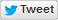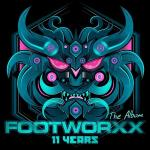0 (0 rates - 0 favorites)

# Al Twisted & Necrotic - 4&3&2&1 lyrics

Four and three and two and one (wooh)
One, two, three, four, come on
[4x]

Four and four and two and one (wooh)
One one, two two, three three, four four
Four and three and two and one (wooh)
One, two, three three, four

Four, three, two and and one (wooh)
One, two, three, four
Four, three, two and one (wooh)
One, two, three three, four
Four and three and two and one...
One, two, three, four!

Four and three and two and one (wooh)
Four and three and two and
One, two, three, four!

We don't know that this universe that we exist in right now
Is the only universe
We don't know whether or not there are infinite universes that exist
They're just now starting to think that inside every black hole
Is another universe

Four and three and two and one (wooh)
One, two, three, four, come on
[4x]

Four and three and two and one...
One, two, three, four!

Four and three and two and
One, two, three, four!
Four and three and two and one (wooh)
One, two, three, four!

Four and three and two and one...
Four, three, two and and one (wooh)
One, two, three, four
Four, three, two and one (wooh)
One, two, three three, four
Four and three and two and one...
One, two, three, four!

Four and three and two and one (wooh)
Four and three and two and
One, two, three, four!

Four and three and two and one (wooh)
One, two, three, four, come on
[2x]

Four and four and two and one (wooh)
One one, two two, three three, four four
Four and three and two and one (wooh)
One, two, three three, four• Footworxx 11 Years - The Album

• Year
• 2014

• Genre

• Source / Sample

• Submitted
• Approved by BlueBerry
• 772 views

Top achievedBETA
#9 Daily Hardcore/Gabber

Comment on this lyric
Register